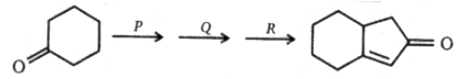Reagent to carry out above conversion,P,Q,R respectively are:

(a) H2C=CH-CH2-Br,(HO$\mathrm{\theta }$),[HO$\mathrm{\theta }$,$∆$], Wacker-process

(b) H2C=CH-CH2-Br(HO$\mathrm{\theta }$), Wacker-process, HO$\mathrm{\theta }$,$∆$

(c) Wacker process, H2C=CH-CH2-Br(HO$\mathrm{\theta }$),HO$\mathrm{\theta }$($∆$)

(d) Wacker process,HO$\mathrm{\theta }$($∆$), H2C=CH-CH2-Br(HO$\mathrm{\theta }$)

Concept Questions :-

Preparation & properties of Aldehydes and Ketones
High Yielding Test Series + Question Bank - NEET 2020

Difficulty Level: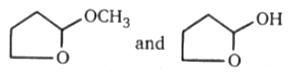Above compounds can be differentiated by following reagent:

(b) Tollen's reagent

(c) Lucas reagent

(d) NaHSO3

Concept Questions :-

Preparation & properties of Aldehydes and Ketones
High Yielding Test Series + Question Bank - NEET 2020

Difficulty Level:Product (D) will be :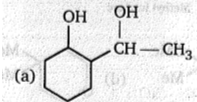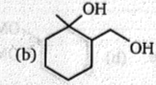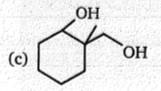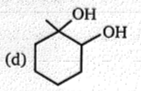Concept Questions :-

Nucleophillic Addition, Reactivity of Alpha Hydrogen in Aldehydes with Uses
High Yielding Test Series + Question Bank - NEET 2020

Difficulty Level: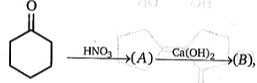Product (B) in this reaction is :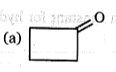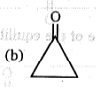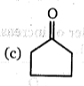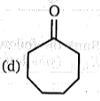Concept Questions :-

Preparation & properties of Aldehydes and Ketones
High Yielding Test Series + Question Bank - NEET 2020

Difficulty Level:

$\mathrm{Ph}-\stackrel{\stackrel{\mathrm{O}}{\mathrm{ll}}}{\mathrm{C}}-{\mathrm{CH}}_{3}\underset{\mathrm{HCl}}{\overset{{\mathrm{NaNO}}_{2}}{\to }}\left(\mathrm{A}\right)\underset{\mathrm{heat}}{\overset{{\mathrm{AC}}_{2}\mathrm{O}}{\to }}\left(\mathrm{B}\right)\stackrel{{\mathrm{H}}_{3}{\mathrm{O}}^{+}}{\to }\left(\mathrm{c}\right)$

Product (C) of the above reaction is :

(a) $\mathrm{Ph}-{\mathrm{CO}}_{2}\mathrm{H}$

(b) $\mathrm{Ph}-\stackrel{\stackrel{\mathrm{O}}{\mathrm{ll}}}{\mathrm{C}}-{\mathrm{CO}}_{2}\mathrm{H}$

(c) $\mathrm{Ph}-\stackrel{\stackrel{\mathrm{O}}{\mathrm{ll}}}{\mathrm{C}}-\stackrel{\stackrel{\mathrm{O}}{\mathrm{ll}}}{\mathrm{C}}-\mathrm{H}$

(d) $\mathrm{Ph}-\stackrel{\stackrel{\mathrm{O}}{\mathrm{ll}}}{\mathrm{C}}-{\mathrm{CO}}_{2}\mathrm{H}$

Concept Questions :-

Preparation & properties of Aldehydes and Ketones
High Yielding Test Series + Question Bank - NEET 2020

Difficulty Level: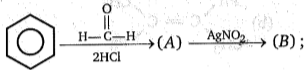Product (B) of the reaction is :

(a) $\mathrm{Ph}-{\mathrm{CH}}_{2}-{\mathrm{NO}}_{2}$

(b) $\mathrm{Ph}-{\mathrm{CH}}_{2}-\mathrm{ONO}$

(c) $\mathrm{Ph}-\mathrm{CHO}$

(d) $\mathrm{Ph}-\mathrm{O}-\mathrm{N}=\mathrm{O}$

Concept Questions :-

Preparation & properties of Aldehydes and Ketones
High Yielding Test Series + Question Bank - NEET 2020

Difficulty Level: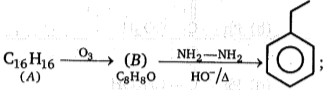Reactant (A) in this reaction is :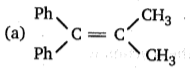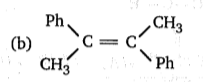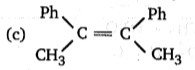(d) Both (b) and (c)

Concept Questions :-

Preparation & properties of Aldehydes and Ketones
High Yielding Test Series + Question Bank - NEET 2020

Difficulty Level:

Correct order of reactivity of following compounds towards Grignard reagent ?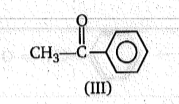(a) I > II >III

(b) II > I > III

(c) II > III > I

(d) I > III > I

Concept Questions :-

Nucleophillic Addition, Reactivity of Alpha Hydrogen in Aldehydes with Uses
High Yielding Test Series + Question Bank - NEET 2020

Difficulty Level:

What reagent and/or reaction conditions would you choose to bring about the following conversion?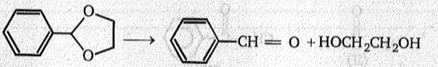(a)

(b)

(c)

(d)

Concept Questions :-

Preparation & properties of Aldehydes and Ketones
High Yielding Test Series + Question Bank - NEET 2020

Difficulty Level: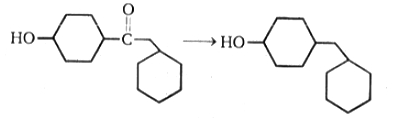The above reduction can be best carried out by:

1. Clemmensen reduction

2. Wolff-Kishner reduction

3. ${\mathrm{NaBH}}_{4}$

4. None of these

Concept Questions :-

Preparation & properties of Aldehydes and Ketones